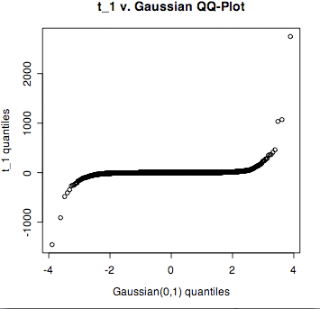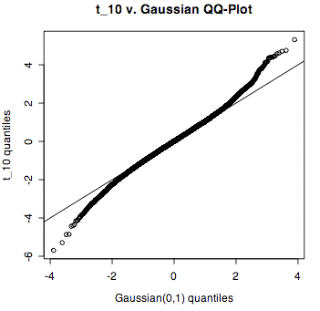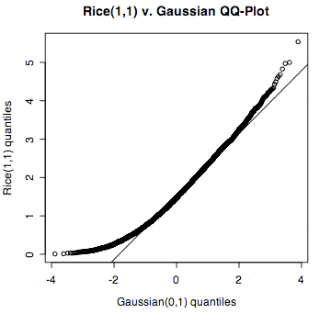Thursday, January 31, 2008

C++ static arrays are initialized every time

The language would be very broken if this wasn't true, but I'm happier having some verification of this anyway:
`#include <iostream>using namespace std;int main(){  for (int i = 0; i < 3; ++i) {    int a = {13};    cout << a << endl;  }  return 0;}`
\$ g++ array_init_every_time.cpp
\$ ./a.out
13
13
13

Wednesday, January 30, 2008

Do I think in closures?

`R:> a <- "Yes"> f <- function() { return(a) }> a <- "No"> f() "No"`
Okay. That's not what I was expecting.
`Scheme:> (define a "Yes")> (define f (lambda () a))> (define a "No")> (f)"No"`
Hm. But (in R):
> f <- (function() {a <- "What?"; list(yes = function() a <- "Yes!", huh = function() cat(a, "\n"))})()
> f\$huh()
What?
> f\$yes()
> f\$huh()
What?

I guess I'll have to take a look at the manual to see exactly what <- means.

Monday, January 28, 2008

Another way to print a matrix in Maxima

I've found Maxima's provided method for printing a matrix as TeX to produce code that isn't usable in LaTeX without a good amount of work. There are apparently conflicts with the more recent version of amsmath. Here're the beginnings of a replacement
`printMatrix(a) := block([as],     as : matrixmap(lambda([x],                      printf(false, "~a", x)), a),     simplode(maplist(lambda([row],                             simplode(row, " & ")),                                         as),              sconc(" ", "\\\\", newline)))\$`
Naturally, again I have to wonder very strongly if there isn't already a better way to do this.

Looking back at diag_matrix, I'm a little embarrassed to see it is in the manual. I could've sworn I looked at all the matches for "diagonal" in the manual.

Sunday, January 27, 2008

Create a diagonal matrix in Maxima

There's got to be a better way to do this! But how?
`diag(d) := block([n], n : length(d),                       genmatrix(lambda([i, j],                          if i = j then d[i] else 0),                              n, n))\$`

---

January 28: Yes, there is a better way! In reply to a question on the Maxima list, Barton Willis pointed out a couple functions: diag in package diag and mat_diag which is included in the environment loaded by default:
`(%i90) diag_matrix(1,2,3);                                  [ 1  0  0 ]                                  [         ](%o90)                            [ 0  2  0 ]                                  [         ]                                  [ 0  0  3 ]`

Saturday, January 26, 2008

Approaching normalityqqnorm(rt(10000, df=1), xlab="Gaussian(0,1) quantiles", ylab="t_1 quantiles", main="t_1 v. Gaussian QQ-Plot")qqnorm(rt(10000, df=10), xlab="Gaussian(0,1) quantiles", ylab="t_10 quantiles", main="t_10 v. Gaussian QQ-Plot")
abline(0, 1)qqnorm(rt(10000, df=100), xlab="Gaussian(0,1) quantiles", ylab="t_100 quantiles", main="t_100 v. Gaussian QQ-Plot")
abline(0, 1)y <- sqrt(rnorm(10000, mean=1)^2 + rnorm(10000)^2)
qqnorm(y, xlab="Gaussian(0,1) quantiles", ylab="Rice(1,1) quantiles", main="Rice(1,1) v. Gaussian QQ-Plot")
qqline(y)y <- sqrt(rnorm(10000, mean=1, sd=10)^2 + rnorm(10000, sd=10)^2)
qqnorm(y, xlab="Gaussian(0,1) quantiles", ylab="Rice(1,100) quantiles", main="Rice(1,100) v. Gaussian QQ-Plot")
qqline(y)

Oh, I've got this backwards. Duh.y <- sqrt(rnorm(10000, mean=100, sd=10)^2 + rnorm(10000, sd=10)^2)
qqnorm(y, xlab="Gaussian(0,1) quantiles", ylab="Rice(100,100) quantiles", main="Rice(100,100) v. Gaussian QQ-Plot")
qqline(y)

But there's more to it than signal-to-noise ratio, it seems. At least the relation between the quantiles doesn't look nearly so linear for Rice(1,1) as it does for Rice(100,100). It doesn't seem surprising that the distance of mu from the origin would play a role, however. This would probably be a good time to break out some math. But it's past my bed time.

C code for reading a CSV file

The world definitely needs another implementation of this.

There are a lot of places where it might be better (more efficient) to keep an extra copy of a value rather looking it up in the struct. I was originally writing this for someone else though and thought it was easier to understand as it is. Maybe I am wrong. Suggestions for improvement are always welcome.

`#include <math.h>#include <stdio.h>#include <stdlib.h>#include <string.h>typedef struct {  int columns;  int rows;  double* data;} Matrix;void freeMatrix(Matrix* aMatrix){  if (aMatrix) {    free(aMatrix->data);    free(aMatrix);  }}int countChar(const char* str, char c){  int count = 0;  while (*str) {    if (*str == c) {      ++count;    }    ++str;  }  return count;}#define MAX_LINE 2000/** * input -- a stream of comma-separated numbers, rows terminated * by new lines. The number of rows is determined from * the first line read. * naValue -- the value that will be used to replace * fields left blank. * * Returns NULL if there is a problem reading from the stream. * Check errno for details. */Matrix* readCsv(FILE* input, double naValue) {  Matrix* result = 0;  char buffer[MAX_LINE];  char* line = fgets(buffer, MAX_LINE, input);  if (!line) {    return 0;  }  else {    result = malloc(sizeof(Matrix));    int total = 0;    int capacity = 0;    result->rows = 0;    result->columns = countChar(line, ',') + 1;    capacity = result->columns * 20;    result->data = malloc(sizeof(double) * capacity);       do {      int i;      if ((total + result->columns) > capacity) { capacity *= 2; result->data = realloc(result->data,                                  sizeof(double) * capacity);      }      for (i = 0; i < result->columns; ++i) { if (!line || ',' == line             || '\n' == line || '\r' == line) {   result->data[total] = naValue; } else {   double value;      if (sscanf(line, "%lf", &value) == 1) {     result->data[total] = value;   }   else {     freeMatrix(result);     return 0;   } } ++total; if (line) {   line = index(line, ',');   if (line) {     ++line;   } }      }      ++(result->rows);      line = fgets(buffer, MAX_LINE, input);    } while (line && ('\n' != line)                     && ('\r' != line));  }    return result;}void printMatrix(FILE* out, const Matrix* aMatrix){  int i;  for (i = 0; i < aMatrix->rows; ++i) {    int j;    const int offset = i * aMatrix->columns;    for (j = 0; j < aMatrix->columns; ++j) {      fprintf(out, "%f ", aMatrix->data[offset + j]);    }    fprintf(out, "\n");  }}int main(int argc, char** argv){  if (argc == 2) {    FILE* in = fopen(argv, "r");    Matrix* m = readCsv(in, -HUGE_VAL);    fclose(in);    if (m) {      printMatrix(stdout, m);      freeMatrix(m);    }    else {      printf("problem reading %s\n", argv);    }  }    return 0;}`

What is with Blogger clipping wide posts? Very helpful! As is inserting BR tags into a textarea. Use some of that magic DHTML to let people fold the sidebar out of the way. (Well, I suppose it's possible for me to add this myself. As newbie I shouldn't be complaining. But I think I am talking to myself now anyway. Hello, you, me!)

Wednesday, January 23, 2008

ifelse(o, a, b)

It should be obvious I guess and is also documented, but something that surprised me: length(ifelse(o, a, b)) == length(o). I encountered this in defining the Rice density in R. I have
`drice <- function(x, mu, sigmaSq) {  ifelse(x >= 0,     x / sigmaSq *      exp(-(x*x + mu*mu)/(2*sigmaSq))*besselI(x*mu/sigmaSq, 0),    0)}`
Now I wanted to look at the density with a fixed x but multiple values of mu. But for instance drice(1, c(1,2), 1) ==> 0.4657596. What's a good way to handle this? It's pretty ugly, but I think this does what I want:
`drice <- function(x, mu, sigmaSq) {  n <- max(length(x), length(mu), length(sigmaSq))  x <- rep(x, length.out=n)  mu <- rep(mu, length.out=n)  sigmaSq <- rep(mu, length.out=n)  ifelse(x >= 0,     x / sigmaSq *      exp(-(x*x + mu*mu)/(2*sigmaSq))*besselI(x*mu/sigmaSq, 0),    0)}`
drice(1, c(1,2), 1) ==> c(0.4657596, 0.1813670) But there has to be a better way.

Tuesday, January 22, 2008

A few string functions for working with case

`##x -- a vector of single element stringscharToUpper <- function(x) {  i <- match(x, letters)  ifelse(!is.na(i), LETTERS[i], x)}##s -- string to be put in uppercaseupper <- function(s) {  paste(charToUpper(strsplit(s, "")[]), collapse="")}titleCase <- function(s) {  n <- nchar(s)  if (n > 0) {    paste(charToUpper(substr(s, 1, 1)),              substr(s, 2, n), sep="")  }  else {    s  }}`
It's hard to believe these aren't defined (in native code) in the base environment. Of course, R's primary focus isn't string manipulation, but these come up naturally enough when building up labels for plots. Maybe they're worried about internationalization issues? (Or maybe I've just missed the relevant functions.)

By the way, the "mystery" over the differences between the mgfs given by C & B for negative binomial and geometric distributions is solved by noting the differences in support (x = 0, 1, ... vs. x = 1, 2, ...) for the two as defined in the book.

Saturday, January 5, 2008

moment generating functions in R

##Binomial
mbinom <- function(t, n, p) (p * exp(t) + (1 - p))^n

##Discrete uniform
mdunif <- function(t, n) apply(exp(t %o% 1:n), 1, sum)

##Geometric
mgeom <- function(t, p) { et <- exp(t); ifelse(t < -log(1 - p), p * et / (1 - (1 - p) * et), NA) }

##Negative binomial
mnbinom <- function(t, p, r) ifelse(t < -log(1 - p), (p / (1 - (1 - p) * exp(t))^r, NA)

##Poisson
mpois <- function(t, r) exp(r * (exp(t) - 1))

##Gamma
mgamma <- function(t, a, b) (1/(1 - b * t))^a

##Normal
mnorm <- function(t, mean, var) exp(mean * t + var * t * t / 2)

##Uniform
munif <- function(t, min, max) (exp(max * t) - exp(min * t)) / ((max - min) * t)

The formulas are copied from Casella and Berger. I haven't checked the code carefully for errors. I'm puzzled why there is an extra exp(t) term in the denominator for the geometric distribution since Geometric(p) = NegBinom(p, r=1). I'll check why later. Now, thank goodness, I'm ready to fall asleep.

Friday, January 4, 2008

an incomplete family

For b > 0, define the pmf p with p(b) = p(-b) = 1/2. Say X ~ p. Now with g(x) = x, Eg(X) = 1/2(b - b) = 0 for all b while P(X = 0) = 0 for all b.